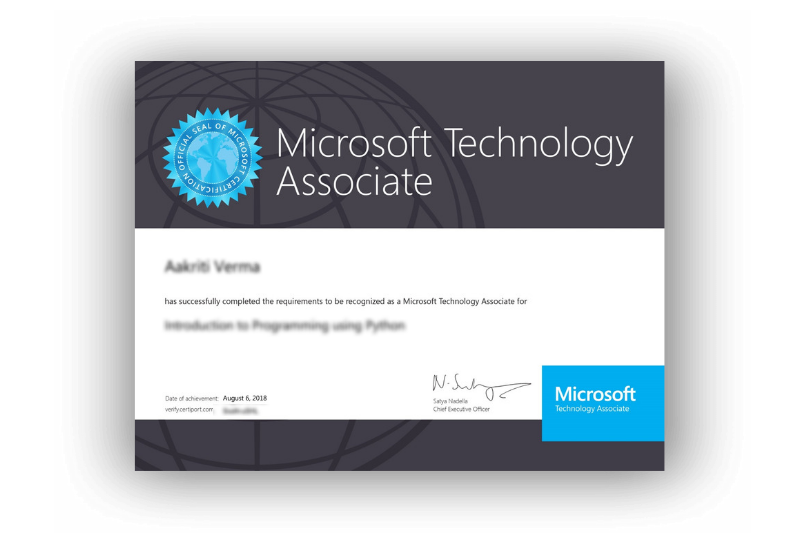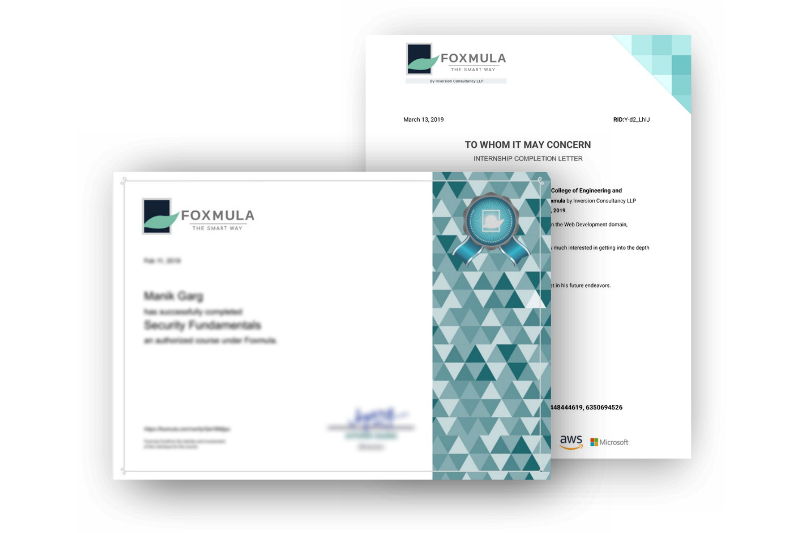# Deep Learning using TensorFlow

## Description

Foxmula’s Deep Learning in TensorFlow course has been designed by industry experts as per the requirements and demands of the industry. This course takes a pragmatic approach to deep learning for professionals active as software developers. During the course, you will learn to build deep learning applications using the TensorFlow. Further, you will get first-hand experience for developing your own highly sophisticated art image classifiers along with other models of deep learning.
The TensorFlow program from us will enable you to learn the fundamental concepts of TensorFlow, its primary functions, about its operations and the execution pipeline. During the duration of the course, you will witness how TensorFlow can be utilized in regression, classification, curve fitting, as well as in minimization of error functions.
Further, you will also be trained to utilize your TensorFlow Models in real world on different mobile devices, in the browsers as well as in the cloud. Lastly, you will learn to use algorithms and advanced techniques to work with datasets of large amount. Upon the completion of the course, you will develop skills and aptitude required to develop your own AI applications.

Course Content –

• What is Machine learning
• Introduction to neural networks
• Basics of Tensor Flow
• Convolutional Neural Network
• Auto Encoders
• Recurrent Neural Networks

What You will Learn during the Course -

• Explain fundamental concepts of TensorFlow such as the main functions, operations as well as the execution pipelines.
• Implementing TensorFlow for classifying the Image using Convolutional Neural Networks
• Learn to create Generative Adversarial Networks using TensorFlow
• Make use of TensorFlow for Classification and Regression Tasks
• Use TensorFlow technique for Time Series Analysis with Recurrent Neural Networks
• Use TensorFlow for the purpose of solving Unsupervised Learning issues using the AutoEncoders

• ###### Training

1 month Training, 6 months access, Industry-Oriented, Self-Paced.

• ###### Certification

Small & basic Objective MCQ type online exams. MTA & Foxmula Certification.

• ###### Internship

45 days Internship Completion letter post project submission on our GitLab. Projects are Industrial, Small and based on your training.## How It Works## Curriculum

Created by the Google Brain team, TensorFlow is an open source library for numerical computation and large-scale machine learning. TensorFlow bundles together a slew of machine learning and deep learning (aka neural networking) models and algorithms and makes them useful by way of a common metaphor. TensorFlow is the most famous library used in production for deep learning models.
Machine learning uses algorithms to parse data, learn from that data, and make informed decisions based on what it has learned. Deep learning structures algorithms in layers to create an “artificial neural network” that can learn and make intelligent decisions on its own.
In machine learning, the perceptron is an algorithm for supervised learning of binary classifiers. A binary classifier is a function which can decide whether or not an input, represented by a vector of numbers, belongs to some specific class.
In deep learning, a convolutional neural network (CNN, or ConvNet) is a class of deep neural networks, most commonly applied to analyzing visual imagery. CNNs use a variation of multilayer perceptrons designed to require minimal pre processing.
A recurrent neural network (RNN) is a class of artificial neural network where connections between nodes form a directed graph along a sequence. This allows it to exhibit temporal dynamic behavior for a time sequence. Unlike feed forward neural networks, RNNs can use their internal state (memory) to process sequences of inputs.
A restricted Boltzmann machine (RBM) is a generative stochastic artificial neural network that can learn a probability distribution over its set of inputs. RBMs were initially invented under the name Harmonium by Paul Smolensky in 1986, and rose to prominence after Geoffrey Hinton and collaborators invented fast learning algorithms for them in the mid-2000.
An autoencoder is a neural network that has three layers: an input layer, a hidden (encoding) layer, and a decoding layer. The network is trained to reconstruct its inputs, which forces the hidden layer to try to learn good representations of the inputs.